# Chemical Engineering - Process Control and Instrumentation

### Exercise :: Process Control and Instrumentation - Section 2

26.

A sinusoidal variation in the input passing through a linear first order system

 A. becomes more oscillatory (frequency increases). B. becomes less oscillatory (frequency decreases). C. gets amplified (magnitude increases). D. gets attenuated (magnitude decreases).

Explanation:

No answer description available for this question. Let us discuss.

27.

Nitrogen gas constant volume thermometer is suitable for measuring a temperature of __________ °C.

 A. 0-100 B. < 0 C. > 100. D. > 50

Explanation:

No answer description available for this question. Let us discuss.

28.

What is the overall transfer function (C/R) of the following block diagram if G = G1. G2. G3 and H = H1.H2.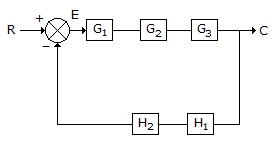A.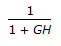B.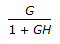C.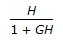D.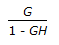Explanation:

No answer description available for this question. Let us discuss.

29.

Pick out the most suitable instrument for measuring temperature in the range of-40 to 425°C.

 A. mercury thermometer B. bimetallic thermometer C. radiation pyrometer D. none of these

Explanation:

No answer description available for this question. Let us discuss.

30.

Accurate temperature measurement performance of a radiation pyrometer can not be affected, if the

 A. enhancement or attentuation of redia-tion occurs in the sighting path. B. object and surrounding are at almost the same temperature. C. object has varying emissivity. D. object is transparent.